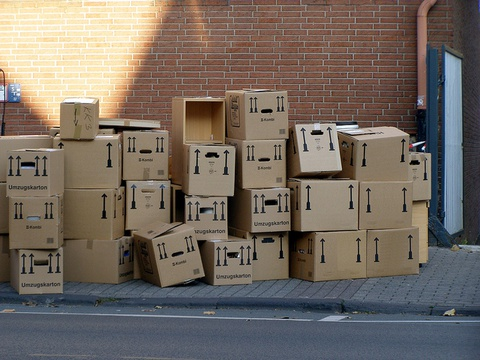# Volume Formula For Rectangular Prisms# Volume of Cubes

The cube at the left of the diagram has edge lengths of 1 unit. The cube at the right has edge lengths of $\frac{1}{4}$ unit.

Volume is the amount of space inside a 3-D figure.

• How many $\frac{1}{4}$-unit cubes fit inside the 1-unit cube?
• What is the volume of the $\frac{1}{4}$-unit cube?1 of 10